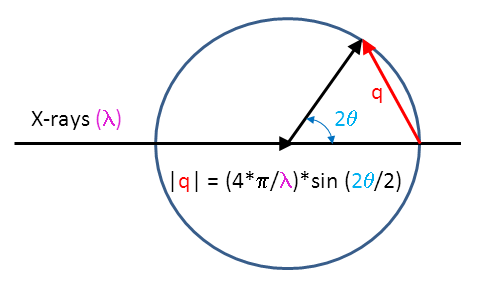Angular conversion between 2*theta and q in small-angle scattering (SAS)

Select either conversion from 'q to 2*theta' or '2*theta to q', the range of conversion and the wavelength. The angular units of 2*theta are in degree (0 -180) and q has the same (but reciprocal) unit as the wavelength. So if the wavelength is given in nm, q will be in inverse nm.

Conversion x range: from to wavelengthAuthor: M.Kriechbaum, TU-Graz (2014), e-mail: manfred.kriechbaum@tugraz.at